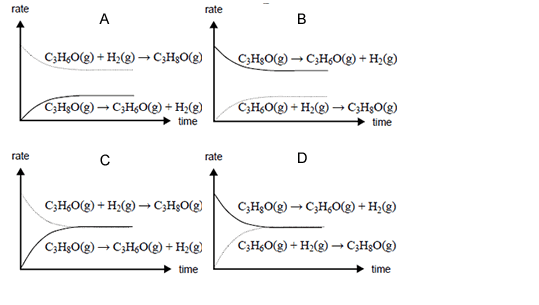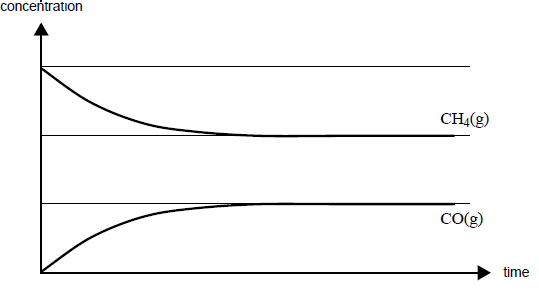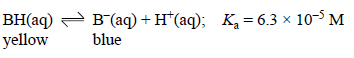Equilibrium chemistry 2007 VCE

Propanone (C3H6O) can be made from 2-propanol using a copper-zinc catalyst.
C3H8O(g) => C3H6O(g) + H2(g); ΔH = 54 kJ /mol

At equilibrium at a particular temperature, 10% of the
2-propanol is converted to propanone.
In order to increase the percentage yield of propanone at equilibrium, you should
A. lower the temperature and lower the pressure.
B. lower the temperature and raise the pressure.
C. raise the temperature and lower the pressure.
D. raise the temperature and raise the pressure.

SolutionWhen 2-propanol reacts to form an equilibrium mixture with propanone and hydrogen, which one of the following
best represents how the rates of the forward and back reactions change over time?

SolutionCarbon monoxide and hydrogen can be produced from the reaction of methane with steam according to the
equation
CH4(g) + H2O(g) => CO(g) + 3H2(g); ΔH = +206 kJ mol1
Some methane and steam are placed in a closed container and allowed to react at a fi xed temperature. The graph on the right shows the change in concentration of methane and carbon monoxide as the reaction progresses.

On the graph, draw a line to show the change in concentration of hydrogen gas as the reaction
progresses. Label this line.

On the graph, draw a line to show how the formation of carbon monoxide would differ over
time in the presence of a catalyst. Label this line.

SolutionGreat care must be taken when handling carbon monoxide as it is a highly poisonous gas. Even at low concentrations it competes very successfully with oxygen for haemoglobin, the oxygen carrier in the blood.
The reactions can be written as
haemoglobin + oxygen => oxyhaemoglobin; K1
haemoglobin + carbon monoxide => carbon monoxidehaemoglobin; K2

What does the above information indicate about the magnitude of the equilibrium constant K1 compared with the magnitude of the equilibrium constant K2?

SolutionThe rates of chemical reactions may be explained using the collision theory model. Indicate whether the following statements about rates and the collision theory model are true or false by placing ticks in the
appropriate boxes.

 Statement Endothermic reactions are always slower than exothermic reactions. True False All particles have the same kinetic energy at a fixed temperature. True False Reactant particles need to collide with sufficient energy to react. True False The rate of a reaction at a constant temperature increases as the reaction proceeds. True False Increasing the temperature increases the fraction of collisions with energy above the activation energy. True False

SolutionBromophenol blue is a weak acid (represented as BH) that acts as an acid-base indicator. In solution the following equilibrium is established.At low pH bromophenol blue exists mainly as the acid, BH, which is yellow in colour, while at high pH it exists mainly as its conjugate base, B, which is blue. An intermediate colour is observed when the concentration of the acid and the concentration of the conjugate base are similar.

Write an expression for Ka in terms of the concentrations of BH, B and H+.

Solution

When [BH] = [B-], the mixture appears green.
Calculate the pH at which [BH] = [B-]

Solution.